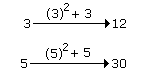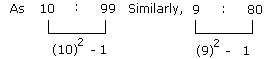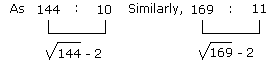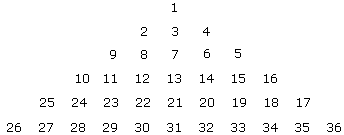Courses

# Test: Analogy- 3

## 20 Questions MCQ Test UPSC CSAT: Logical Reasoning (LR) & Data Interpretation (DI) | Test: Analogy- 3

Description
This mock test of Test: Analogy- 3 for SSC helps you for every SSC entrance exam. This contains 20 Multiple Choice Questions for SSC Test: Analogy- 3 (mcq) to study with solutions a complete question bank. The solved questions answers in this Test: Analogy- 3 quiz give you a good mix of easy questions and tough questions. SSC students definitely take this Test: Analogy- 3 exercise for a better result in the exam. You can find other Test: Analogy- 3 extra questions, long questions & short questions for SSC on EduRev as well by searching above.
QUESTION: 1

### Directions to Solve In each of the following questions find out the alternative which will replace the question mark. Question - 14 : 9 :: 26 : ?

Solution:

14 = (2 x 9 - 4)

26 = (2 x 15 - 4)

? = 15

QUESTION: 2

### Directions to Solve In each of the following questions find out the alternative which will replace the question mark. Question - MO : 13 11 :: HJ : ?

Solution:

Count the alphabets in reverse from z.
m is 13th, o is 11th,
and 18th n j is 16th

QUESTION: 3

### Directions to Solve In each of the following questions find out the alternative which will replace the question mark. Question - 123 : 132 :: 235 : ?

Solution:

As, 123 → 132

As, 235 → 253

The middle digit of first term becomes power to the next term.

QUESTION: 4

Directions to Solve

In each of the following questions find out the alternative which will replace the question mark.

Question -

8 : 28 :: 27 : ?

Solution:

First number = 8 and the sum of the digits of the second number is 2 + 8 = 10.

Thus the difference of the first number and the sum of the digits of second number is 10 - 8 = 2.

Similarly, the sum of the digits of third number is 2 + 7 = 9.

Hence the sum of digits of fourth number should be 2 more than 9 i.e. 11

Hence, fourth number is 65.

QUESTION: 5

Directions to Solve

In each of the following questions find out the alternative which will replace the question mark.

Question -

3 : 12 :: 5 : ?

Solution:QUESTION: 6

Directions to Solve

In each of the following questions find out the alternative which will replace the question mark.

Question -

MXN : 13 x 14 :: FXR : ?

Solution:

As position of M and N in Eq. alphabets are 13 and 14 respectively.

QUESTION: 7

Directions to Solve

In each of the following questions find out the alternative which will replace the question mark.

Question -

16 : 56 :: 32 : ?

Solution:

As, 16:56 = (2/7)

Similarly, 32:112:(2/7)

QUESTION: 8

Directions to Solve

In each of the following questions find out the alternative which will replace the question mark.

Question -

4 : 19 :: 7 : ?

Solution:

As, (4)2 + 3 = 19

Similarly, (7)2 + 3 = 52

QUESTION: 9

Directions to Solve

In each of the following questions find out the alternative which will replace the question mark.

Question -

24 : 60 :: 120 : ?

Solution:

As 24:60 = (2/5)

Similarly, (120/300) = (2/5)

QUESTION: 10

Directions to Solve

In each of the following questions find out the alternative which will replace the question mark.

Question -

335 : 216 :: 987 : ?

Solution:

As 335 - 216 = 119

Similarly, 987 - X = 119

Therefore, X = 987 - 119 = 868.

QUESTION: 11

Directions to Solve

In each of the following questions find out the alternative which will replace the question mark.

Question -

10 : 99 :: 9 : ?

Solution:QUESTION: 12

Directions to Solve

In each of the following questions find out the alternative which will replace the question mark.

Question -

8 : 24 :: ? : 32

Solution:

24 → 2 x 4 = 8

32 → 3 x 2 = 6

QUESTION: 13

Directions to Solve

In each of the following questions find out the alternative which will replace the question mark.

Question -

144 : 10 :: 169 : ?

Solution:QUESTION: 14

Directions to Solve

In each of the following questions find out the alternative which will replace the question mark.

Question -

9 : 8 :: 16 : ?

Solution:

9 = (3)2

8 = (3 - 1)3

and 16 = (4)2

? = (4 - 1)3 = 27

QUESTION: 15

Directions to Solve

In each of the following questions find out the alternative which will replace the question mark.

Question -

In this pyramid number if: 11 22 31 : 12 21 32 :: 9 12 21 : ?Solution:

If we see diagonally we will get 11 22 31 and the next diagonal series is 12 21 32. Similarly the next diagonal series after 9 12 21 is 8 13 20.

QUESTION: 16

Directions to Solve

In each of the following questions find out the alternative which will replace the question mark.

Question -

25 : 37 :: 49 : ?

Solution:

As 25 = (5)2 → (5 + 1)2 + 1 = 37.

As 49 = (7)2 → (7 + 1)2 + 1 = 65.

QUESTION: 17

Directions to Solve

In each of the following questions find out the alternative which will replace the question mark.

Question -

K/T : 11/20 :: J/R : ?

Solution:

In Eq. alphabets positions of K and T are 11 and 20 respectively.

Similarly positions of J and R are 10 and 18.

QUESTION: 18

Directions to Solve

In each of the following questions find out the alternative which will replace the question mark.

Question -

68 : 130 :: ? : 350

Solution:

As, 68 = (4)3 + 4

130 = (5)3 + 5

and 350 = (7)3 + 7

Therefore, ? = (6)3 + 6 = 222

QUESTION: 19

Directions to Solve

In each of the following questions find out the alternative which will replace the question mark.

Question -

61 : 121 :: ? : 337

Solution:

As, 61 = (4)3 - 3

121 = (5)3 - 4

and 337 = (7)3 - 6

Therefore, ? = (6)3 - 5 = 211

QUESTION: 20

Directions to Solve

In each of the following questions find out the alternative which will replace the question mark.

Question -

27 : 125 :: 64 : ?

Solution:

27 → 33; 125 → 53; 64 → 43

Therefore ? = 63 = 216.